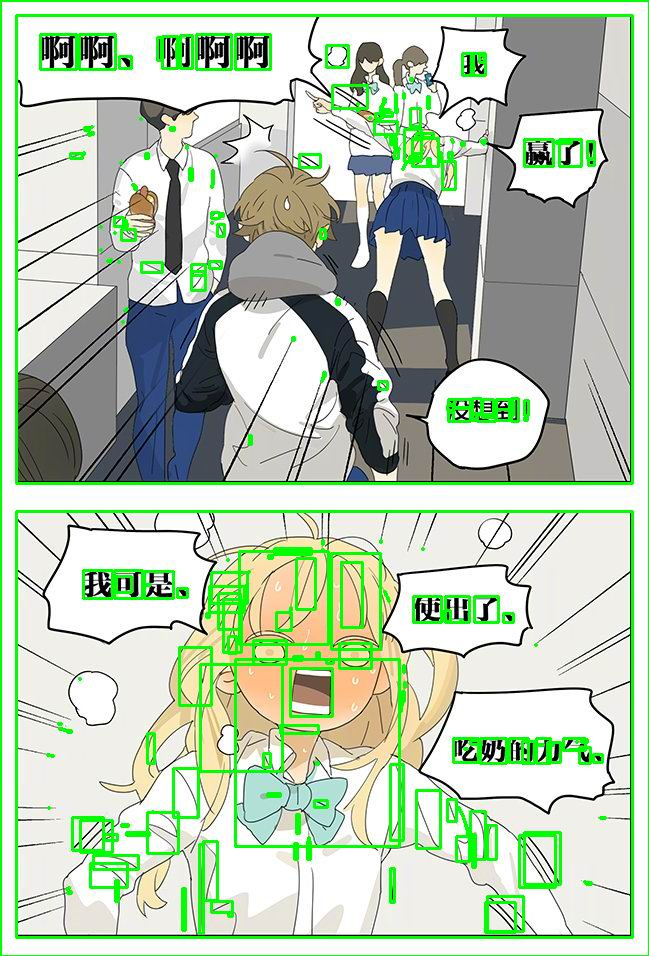# K-Means聚类及在漫画中的应用

| 分类 技术随笔  | 标签 图像处理

K-Means聚类需要用户指定K个分类，取随机K个种子点（质心），跟种子点近的数据可以归为一类。之后重新确定质心，看和原来的质心差别是不是不大，不大则大致可以完成聚类。import cv2
import numpy as np

gray = cv2.cvtColor(img, cv2.COLOR_BGR2GRAY)
thresh = cv2.threshold(gray, 0, 255, cv2.THRESH_BINARY_INV + cv2.THRESH_OTSU)
_, labels, stats, centroids = cv2.connectedComponentsWithStats(thresh)

X=[]

for stat in stats:
h=stat[cv2.CC_STAT_HEIGHT]
one=[] #单个数据点，可以添加多个数据
one.append(h)
X.append(one)

kmeans_model = KMeans(n_clusters=3) #设置分类为3
y_pred= kmeans_model.fit_predict(X)
print(y_pred) #输出分类结果

index=0

#不同分类设置不同颜色

color1=(255,0,0)
color2=(0,255,0)
color3=(0,0,255)

cls1=[]
cls2=[]
cls3=[]

for cls in y_pred:
if cls==0:
color=color1
cls1.append(stats[index])
elif cls==1:
color=color2
cls2.append(stats[index])
else:
color=color3
cls3.append(stats[index])

stat=stats[index]
x=stat[cv2.CC_STAT_LEFT]
y=stat[cv2.CC_STAT_TOP]
w=stat[cv2.CC_STAT_WIDTH]
h=stat[cv2.CC_STAT_HEIGHT]
cv2.rectangle(img, (x, y), (x + w, y + h), color, 2)
index=index+1

cv2.imwrite('labeled.png', img)
cv2.namedWindow("labeled.png",cv2.WINDOW_NORMAL)
cv2.imshow('labeled.png', img)
cv2.waitKey()